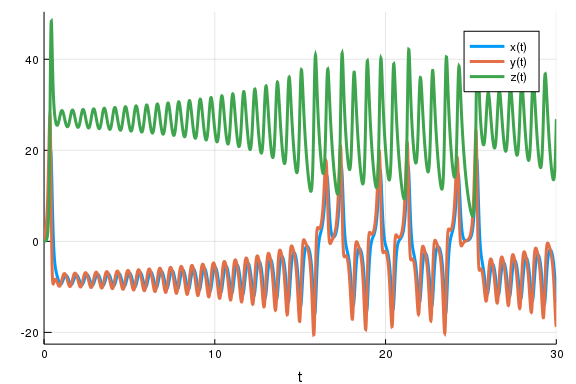# Lorenz Bayesian Parameter Estimation Benchmarks

## Parameter estimation of Lorenz Equation using DiffEqBayes.jl

using DiffEqBayes

loaded

using Distributions
using OrdinaryDiffEq, RecursiveArrayTools, ParameterizedFunctions
using Plots

gr(fmt=:png)

Plots.GRBackend()


#### Initializing the problem

g1 = @ode_def LorenzExample begin
dx = σ*(y-x)
dy = x*(ρ-z) - y
dz = x*y - β*z
end σ ρ β

(::Main.WeaveSandBox0.LorenzExample{getfield(Main.WeaveSandBox0, Symbol("##
1#5")),getfield(Main.WeaveSandBox0, Symbol("##2#6")),getfield(Main.WeaveSan
dBox0, Symbol("##3#7")),Nothing,Nothing,getfield(Main.WeaveSandBox0, Symbol
("##4#8")),Expr,Expr}) (generic function with 2 methods)

r0 = [1.0; 0.0; 0.0]
tspan = (0.0, 30.0)
p = [10.0,28.0,2.66]

3-element Array{Float64,1}:
10.0
28.0
2.66

prob = ODEProblem(g1,r0,tspan,p)
sol = solve(prob,Tsit5())

retcode: Success
Interpolation: specialized 4th order "free" interpolation
t: 357-element Array{Float64,1}:
0.0
3.5678604836301404e-5
0.0003924646531993154
0.0032623362978895253
0.00905769384828584
0.016955582524407417
0.02768842697456643
0.04185394003674388
0.060236921553619587
0.08368074642983417
⋮
29.43765805702477
29.510631239565896
29.578880567314734
29.659017275004008
29.727496214049776
29.810471259396742
29.90700141130956
29.993678718729086
30.0
u: 357-element Array{Array{Float64,1},1}:
[1.0, 0.0, 0.0]
[0.999643, 0.000998805, 1.78143e-8]
[0.996105, 0.0109654, 2.14696e-6]
[0.96936, 0.0897687, 0.000143797]
[0.924207, 0.242279, 0.0010461]
[0.880049, 0.438715, 0.00342406]
[0.848333, 0.691528, 0.0084873]
[0.8495, 1.01449, 0.0182119]
[0.913889, 1.44248, 0.0366944]
[1.08882, 2.05219, 0.074029]
⋮
[-7.11525, -1.1001, 32.1527]
[-3.6978, -0.426863, 26.6792]
[-2.22809, -1.03217, 22.3688]
[-1.89224, -2.12005, 18.2938]
[-2.36411, -3.51716, 15.6141]
[-3.88697, -6.52388, 13.6665]
[-7.69711, -13.0948, 15.3928]
[-12.9863, -18.6942, 25.7278]
[-13.3371, -18.7185, 26.8431]


#### Generating data for bayesian estimation of parameters from the obtained solutions using the Tsit5 algorithm by adding random noise to it.

t = collect(linspace(1,30,30))

ERROR: UndefVarError: linspace not defined

sig = 0.49
data = convert(Array, VectorOfArray([(sol(t[i]) + sig*randn(3)) for i in 1:length(t)]))

ERROR: UndefVarError: t not defined


#### Plots of the generated data and the actual data.

Plots.scatter(t, data[1,:],markersize=4,color=:purple)

ERROR: UndefVarError: data not defined

Plots.scatter!(t, data[2,:],markersize=4,color=:yellow)

ERROR: UndefVarError: data not defined

Plots.scatter!(t, data[3,:],markersize=4,color=:black)

ERROR: UndefVarError: data not defined

plot!(sol)#### Uncertainity Quantification plot is used to decide the tolerance for the differential equation.

cb = AdaptiveProbIntsUncertainty(5)

ERROR: UndefVarError: AdaptiveProbIntsUncertainty not defined

monte_prob = MonteCarloProblem(prob)
sim = solve(monte_prob,Tsit5(),num_monte=100,callback=cb,reltol=1e-5,abstol=1e-5)

ERROR: UndefVarError: cb not defined

plot(sim,vars=(0,1),linealpha=0.4)

ERROR: UndefVarError: sim not defined

cb = AdaptiveProbIntsUncertainty(5)

ERROR: UndefVarError: AdaptiveProbIntsUncertainty not defined

monte_prob = MonteCarloProblem(prob)
sim = solve(monte_prob,Tsit5(),num_monte=100,callback=cb,reltol=1e-6,abstol=1e-6)

ERROR: UndefVarError: cb not defined

plot(sim,vars=(0,1),linealpha=0.4)

ERROR: UndefVarError: sim not defined

cb = AdaptiveProbIntsUncertainty(5)

ERROR: UndefVarError: AdaptiveProbIntsUncertainty not defined

monte_prob = MonteCarloProblem(prob)
sim = solve(monte_prob,Tsit5(),num_monte=100,callback=cb,reltol=1e-8,abstol=1e-8)

ERROR: UndefVarError: cb not defined

plot(sim,vars=(0,1),linealpha=0.4)

ERROR: UndefVarError: sim not defined

priors = [Truncated(Normal(10,2),1,15),Truncated(Normal(30,5),1,45),Truncated(Normal(2.5,0.5),1,4)]

3-element Array{Distributions.Truncated{Distributions.Normal{Float64},Distr
ibutions.Continuous},1}:
Truncated(Distributions.Normal{Float64}(μ=10.0, σ=2.0), range=(1.0, 15.0))
Truncated(Distributions.Normal{Float64}(μ=30.0, σ=5.0), range=(1.0, 45.0))
Truncated(Distributions.Normal{Float64}(μ=2.5, σ=0.5), range=(1.0, 4.0))


## Parameter estimation using Stan.jl backend.

Lorenz equation is a chaotic system hence requires very low tolerance to be estimated in a reasonable way, we use 1e-8 obtained from the uncertainity plots. Use of Truncated priors is necessary to prevent Stan from stepping into negative and other improbable areas.

@time bayesian_result = stan_inference(prob,t,data,priors;reltol=1e-8,abstol=1e-8,vars=(StanODEData(),InverseGamma(3,2)))

ERROR: UndefVarError: t not defined

plot_chain(bayesian_result)

ERROR: UndefVarError: bayesian_result not defined


### Parameter estimation using Turing.jl backend

@time bayesian_result_turing = turing_inference(prob,Tsit5(),t,data,priors)

ERROR: UndefVarError: t not defined

plot_chain(bayesian_result_turing)

ERROR: UndefVarError: bayesian_result_turing not defined


## Conclusion

Due to the chaotic nature of Lorenz Equation, it is a very hard problem to estimate as it has the property of exponentially increasing errors. Its uncertainity plot points to its chaotic behaviour and goes awry for different values of tolerance, we use 1e-8 as the tolerance as it makes its uncertainity small enough to be trusted in (0,30) time span.

The behaviour is estimation using Stan.jl backend is as expected and it gives more accurate results as we decrease the tolerance, for 1e-8 we obtain quite accurate results as compared to higher tolerance values but lowering the tolerance leads to longer sampling time, incase of 1e-8 it took 11 hours. We also pass 500 warmup samples for proper convergence, as the plots provide evidence of non-convergence without it which observed over multiple runs.